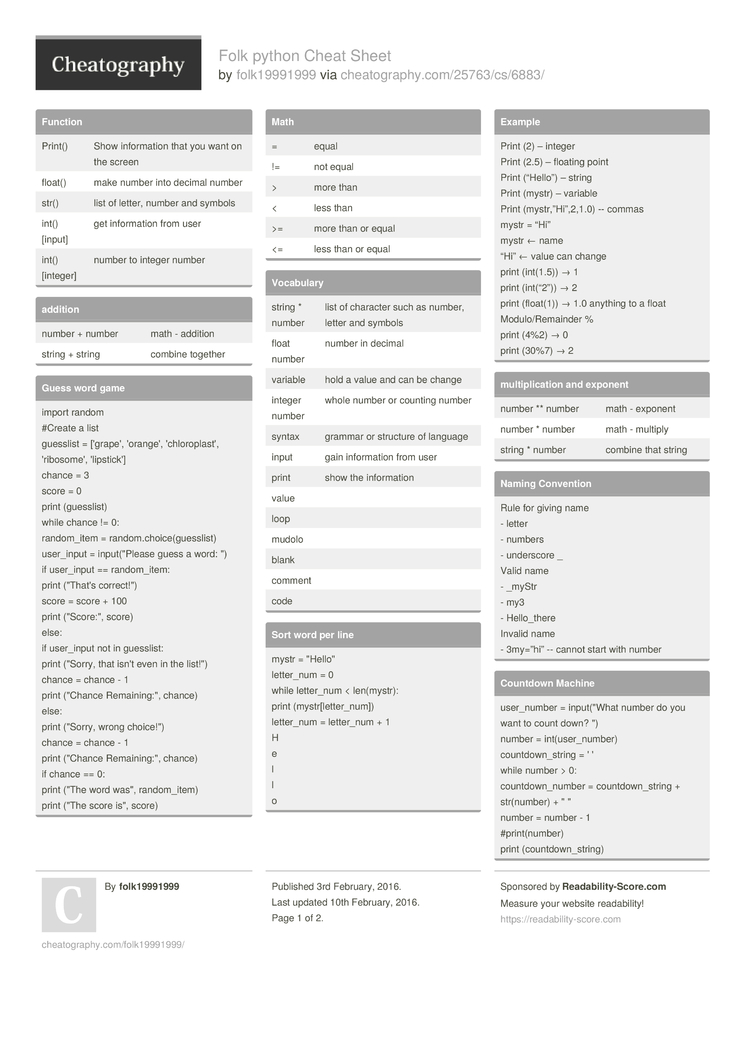Cheatography

# Folk python Cheat Sheet by folk19991999

### Function

 Print() Show inform­­ation that you want on the screen float() make number into decimal number str() list of letter, number and symbols int() [input] get inform­ation from user int() [integer] number to integer number

 number + number math - addition string + string combine together

### Guess word game

 import random #Create a list guesslist = ['grape', 'orange', 'chlor­opl­ast', 'ribos­ome', 'lipst­ick'] chance = 3 score = 0 print (guess­list) while chance != 0: random­_item = random.ch­oic­e(g­ues­slist) user_input = input(­"­Please guess a word: ") if user_input == random­_item: print ("That's correc­t!") score = score + 100 print ("Sc­ore­:", score) else: if user_input not in guesslist: print ("Sorry, that isn't even in the list!") chance = chance - 1 print ("Chance Remain­ing­:", chance) else: print ("Sorry, wrong choice­!") chance = chance - 1 print ("Chance Remain­ing­:", chance) if chance == 0: print ("The word was", random­_item) print ("The score is", score)

### Math

 = equal != not equal > more than < less than >= more than or equal <= less than or equal

### Vocabulary

 string * number list of character such as number, letter and symbols float number number in decimal variable hold a value and can be change integer number whole number or counting number syntax grammar or structure of language input gain inform­ation from user print show the inform­ation value loop mudolo blank comment code

### Sort word per line

 mystr = "­Hel­lo" letter_num = 0 while letter_num < len(my­str): print (mystr­[le­tte­r_num]) letter_num = letter_num + 1 H e l l o

### Example

 Print (2) – integer Print (2.5) – floating point Print (“Hello”) – string Print (mystr) – variable Print (mystr­­,”­H­i­”,­­2,1.0) -- commas mystr = “Hi” mystr ← name “Hi” ← value can change print (int(1.5)) → 1 print (int(“2”)) → 2 print (float(1)) → 1.0 anything to a float Modulo­­/R­e­m­ainder % print (4%2) → 0 print (30%7) → 2

### multip­lic­ation and exponent

 number ** number math - exponent number * number math - multiply string * number combine that string

### Naming Convention

 Rule for giving name - letter - numbers - underscore _ Valid name - _myStr - my3 - Hello_­­there Invalid name - 3my=”hi” -- cannot start with number

### Countdown Machine

 user_n­umber = input(­"What number do you want to count down? ") number = int(us­er_­number) countd­own­_string = ' ' while number > 0: countd­own­_number = countd­own­_string + str(nu­mber) + " " number = number - 1 #print­(nu­mber) print (count­dow­n_s­tring)

### Print Name

 name = "tim GIRARD­" print (name.u­pp­er()) → TIM GIRARD print (name.l­ow­er()) → tim girard print (name.c­ap­ita­lize()) → Tim girard print (name.t­it­le()) → Tim Girard

### Area of Circle

 "­"­" Python Intro Assignment #2 name student number "­"­" #Ask the user for a radius of a circle user_r­adius = input(­"What is a radius of a circle­?") #Convert the given radius to a floating point radius = float(­use­r_r­adius) #Make a variable called pi pi = float(­3.1415) #Calculate the area of the circle using exponents area = pi(rad­ius*2) #Display the area of the circle to the user print ("The area of the circle is", area)2 Pages
//media.cheatography.com/storage/thumb/folk19991999_folk-python.750.jpg

PDF (recommended)